Working Draft 15-May-1997

# 4. Content Markup

## 4.1 Introduction

### 4.1.1 The Intent of Content Markup

As has been noted in the introductory sections of this report, Mathematics can be distinguished by its use of a (relatively) formal language, mathematical notation. Mathematics and its notation should not be viewed as one and the same thing. The intent of content markup in HTML maths is to support the encoding of underlying mathematical content of an expression, rather than any particular rendering for the expression.

For example the construct "H multiplied by e" is expressed using an explicit operator H<TIMES/>e. In different presentational contexts, the multiplication operator might be invisible (e.g. on paper), or rendered as the spoken word "times". Knowing the underlying mathematical construct, it is often possible to generate many different presentations according to the context and style preferences of the author or reader. For common expressions a default visual presentation is usually clear. "Take care of the sense and the sounds will take care of themselves" wrote Lewis Carroll [LC1]. Going in the reverse direction, that is from a presentation form to the underlying construct, is not necessarily easy: "He" could be interpreted as an atomic text string (or a chemical symbol). Context information may be required to decide between possible interpretations. Clues as to the interpretation are plainly important to speech rendering.

Mathematical presentation changes with culture and time: some expressions in combinatorial mathematics today would have one meaning to an English mathematician, and quite another to a French mathematician. Notations may lose currency, for example the use of musical sharp and flat symbols to denote minima and maxima. [TWC1] A notation in use in 1644 for the multiplication mentioned above was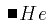.[Cajori]

Encoding the underlying mathematical constructs allows us to better interchange information with systems which are able to manipulate the mathematics. In the trivial example above, such a system could substitute values for the variables H and e, evaluating the result. Further interesting application areas include CD-based textbooks and other interactive teaching aids.

### 4.1.2 The Scope of Content Markup

It is clear that the semantics of much mathematical notation is not yet a matter of consensus. In any case it would be an enormous job to do to codify most of mathematics. Therefore, the MathML proposal specifies a number of commonplace mathematical constructs which should be useful to a large number of potential users. The content tags set out below should be adequate for simple coding of most of the formulas used from kindergarten to the end of high school in the US, and probably beyond through the first two years of college, that is up to A-Level or Baccalaureat level.

The areas covered to some extent in this initial draft are:

• Algebra
• Calculus
• Set Theory
• Series
• Trigonometry
• Statistics
• Matrices

It is not claimed, or even suggested, that the proposed element set is complete for these areas.

### 4.1.3 Basic Concepts of Content Markup

The guidelines governing the design of the MathML content elements are the following principles:

• Expression tree structure of mathematical expressions should be directly encoded by MathML content tags.
• The encoding of simple mathematical expressions should be as natural as possible.
• The encoding of an expression tree should be explicit, and not dependent on additional processing such as operator precedence parsing.

In order to accomplish these goals, MathML introduces two kinds of content tags. The first kind function as containers, and in general serve to mark the scope of the operators contained in them, or to define a context for the elements contained in them, such as a matrix. The second kind are empty elements, such as <SIN/>, and typically represent operators and functions. Operators and functions are applied to arguments by using another empty element, the <APPLY> element.

While MathML content tags do directly encode mathematical meanings, they do not directly control the notation used to present the meaning to a reader. However, content tags have recommended default visual renderings as described in comments where appropriate. In addition, all content tags have a "LAYOUT" attribute (see Section sec4.1.4, which can be used to pass rendering preference information on to a specific renderer which can make use of it.

#### The <EXPR> Construct

The basic building block of a mathematical expression in MathML content markup is the EXPR element. An EXPR corresponds to a complete mathematical expression. Roughly speaking, this means a piece of mathematics which could be surrounded by parentheses or "logical brackets" without changing its meaning.

For example,might be encoded as

<EXPR> x <PLUS/> y </EXPR>.

Since the bracketing is logical, it need not necessarily be rendered. By default, an EXPR is rendered using the MROW presentation schema. Authors can further control how an EXPR is rendered by mixing presentation and content tags (for example adding parentheses), or by using the LAYOUT attribute with a specific renderer.

Using EXPR, (as with braces in traditional mathematics notation), it is possible to specify exactly the scope of any operator or function. The content model of EXPR is simple and recursive. Symbolically, the content model can the described as:

 EXPR => a op b 
where a and b are simple identifiers, or EXPR constructs themselves, and op is any operator or function. Note that this allows EXPR constructs to be nested to arbitrary depth.

An EXPR may also optionally contain more than one operator:

 EXPR => a op b [op c ...] 
For example,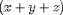can be encoded as
 <EXPR> x <PLUS/> y <PLUS/> z </EXPR>. 

When an EXPR is used in this way, it is important to keep in mind the issue of operator precedence. In cases where several operators are enclosed in a single EXPR, operator association or precedence must be resolved by an external processing application, if it wishes to evaluate the EXPR. Therefore, in most situations, it is probably preferable to fully bracket expressions, particularly when several different operators are involved. For example,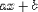is better encoded as

 <EXPR><EXPR>a<TIMES/>x</EXPR> <PLUS/>b</EXPR> 
although it is also valid to encode it as
 <EXPR>a<TIMES/>x<PLUS/>b</EXPR>. 

In addition to determining the scope of operators and functions, the <EXPR> container plays an important role in grouping expressions within other constructs. For example, by default a <VECTOR> element expects to have its components separated by an explicit separator, the <SEP/> tag. However, an expression enclosed by an EXPR is viewed as a single coherent molecule, so that the <SEP/> tag is not needed to separate it from its neighbors.

#### The APPLY construct

One reason for using MathML content markup is to make the mathematical expressions easily available to external processing applications such as computer algebra systems, and intelligent renderers. Therefore, a key requirement of functional representation in content markup is that it must be possible to perform symbolic algebra on mathematical functions as first class objects, and the apply the result to an argument. That is, in addition to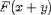we must be able to encode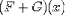.

To understand the MathML approach to applying a function to an argument, consider f(x). This can be encoded as

 <EXPR>f <APPLY/> x </EXPR> 
Note that f and x may be simple identifiers, or more complex constructs built from EXPRs and other function elements. For example, one can construct 'new' functions which can then be applied to an argument using the APPLY element. Thus, the expression (F + G)-1(x) can be encoded as
 <EXPR> <EXPR><INVERSE/><APPLY/><EXPR>F <PLUS/> G</EXPR></EXPR><APPLY/>x </EXPR> 

Most functions supported explicitly in MathML are all canonically empty elements and can be used as first class objects. Examples are <PLUS/>, <SIN/> and user defined functions such as <FN NAME="F"/>. Although, it is probably best to always use the APPLY element, just as it is prudent to fully bracket expressions with EXPRs, the APPLY element is optional and may be omitted in unambiguous situations. The only such situation which commonly arises is when a function named in MathML appears alone with an argument in an EXPR. For example, we can write sin (x) as

 <EXPR><SIN/>x </EXPR> 
as there is no other operator within the EXPR.

There is no linguistic difference in MathML between operators and functions. The separation in the content model is only to clarify what is going on. Some functions in this list may not ever be used in symbolic manipulations.

By default, the APPLY element renders as a thin space between the function and its argument, or as the spoken word "of". Note that the default rendering of the APPLY element does not determine whether or not there are parentheses around the function argument. An author can control this by mixing content and presentation tags, or by using the LAYOUT attribute with a specific renderer.

#### The INVERSE construct

The INVERSE construct is problematic from a mathematical point of view in that it implicitly involves the definition of an inverse for an arbitrary function F. Even at the K through 12 level the concept of an inverse F-1 of many common functions F is not used in a uniform way. For example, inverse trigonometric functions are inverses in a slightly different way than the log function is the inverse of the exponential.

In an effort to be as inclusive as possible, MathML adopts the view that

"If F is a function from a domain D to D', then the inverse G of F is a function over D' such that G(F(x)) = x for x in D."
This definition does not assert that such an inverse exists for all or indeed any x in D, or that it is single-valued anywhere. Authors writing pedagogical material that may be evaluated by other applications may therefore wish to address the issue of the existence of a particular inverse function.

### 4.1.4 Content Markup Layout

Content or semantic tagging goes along with the (frequently unconscious) premise that, if you know the semantics, you can always work out a presentation form. When an author's main goal is to mark up re-usable, evaluatable mathematical expressions, the exact rendering of the expression probably doesn't matter, provided it is easily understandable. However, when an author's goal is more along the lines of providing enough additional semantic information to make a document more accessible by facilitating better visual rendering, voice rendering, or specialized processing, controlling the exact notation used becomes more of an issue.

To help provide authors with more control over layout, every MathML content tag accepts a "LAYOUT" attribute. The "LAYOUT" attribute is there for the author to express a preference between equivalent forms for a particular construct, where the selection of the presentation has nothing to do with the semantics. Examples might be

• inline or displayed equations
• scriptstyle fractions
• use of x with a dot for a derivative over dx/dt

The information provided in the "LAYOUT" attribute is intended for use by specific renderers, and therefore, the valid values are determined by the renderer being used. It is legal for a renderer to ignore this information. This might be intentional, in the case of a publisher imposing a house style, or simply because the renderer does not understand them, or is unable to carry them out.

As a proof of concept, Stilo Technologies have developed a translator which takes as input mathematical formalism encoded in the SGML semantic maths DTD, and generates print-quality TeX. The only presentation information is in the layout attribute, which is used to select the first two options above. This is not a commercial product, but it does enough to validate the concept. There will be a need for the construction of translators for the layout of material tagged with content tags according to the wishes of those wanting the output.

To assist developers wishing to implement a MathML compliant renderer, we give a few suggested default layout rules for rendering MathML content tags via MathML presentation tags. This list is far from complete, but should give an idea of what is intended. Of course, developers are free to use any collection of default rules, provided that they generate an easily understandable rendering for any valid MathML expression.

1. Naked arguments which are numerical (i.e. consist of digits, an optional decimal point, and an optional preceding minus sign) should be treated as though they are wrapped in <MN> tags
2. The characters []{}() and | should be treated as though they are wrapped in <MF> tags when they appear as naked data.
3. Other naked arguments should be treated as though they are wrapped in <MI> tags.
4. <EXPR> pairs are treated as invisible grouping brackets, like the braces of TeX; for presentation they are mapped into <MROW> pairs. If you want a group to be displayed within parentheses or brackets, then you will have to add this explicitly. It is to be expected that, for instance, a parenthesis pair added for this purpose, as, say, in <EXPR><SIN/>(x)</EXPR> will be thrown away as redundant by a semantically oriented further processor.
5. A <SET> container should just provide braces around whatever is within it. The <ST/> should be rendered with a vertical bar.
6. A <MATRIX> container should provide parentheses around whatever is within it, and its subexpressions should be mapped onto an equivalent <MTABLE> construct.
7. <INT> containers should map onto the corresponding expressions in the schema:
<MROW>
<MSUBSUP>
<MO>∫<MO>
< ... LOWLIMIT ...>
< ... UPLIMIT ...>
<MSUBSUP>
<MROW>
<MROW>
... contents ...
</MROW>
<MO>&InvisibleTimes<MO>
<MROW>
<MO>ⅆ<MO>
< ... BVAR ... >
</MROW>
</MROW>
</MROW>

8. <SUM> and <PRODUCT> containers are treated in a way analogous to <INT>.

## 4.2 The Content Markup Elements

The MathML content tags are given in tables below. They are grouped in categories which roughly reflect their grouping in the MathML DTD.

### 4.2.1 Basic Content Schema

TagDescriptionSample Rendering
<APPLY/> makes explicit application of a function to its argument
<E> equation; (see notes)<EA> equation array; (see notes)
<EA>
<E> x <EQ/> 3 </E>
<E> y <EQ/> z <EQ/> w </E>
</EA><EXPR> "scoping" or "bracketing" element
<FN> user-defined function; (see notes)
<FN NAME="F">

<INTERVAL> interval constructor; (see notes)
<INTERVAL CLOSURE="Open-Closed">
a<SEP/>b
</INTERVAL>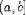<INVERSE> generic inverse for functions
<SEP/> generic separator; (see notes)
<ST/> "such that" separator; (see notes)
<SET>
i <ST./>
<E>1<LE>i<LE>n</E>
</SET>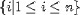#### Notes on Basic Content Schema:

E and EA
The reason for using an explicit equation identifier <E> or equation array identifier <EA> is that it may be used by an automatic equation numbering device, or for indexing, say. In each case there is an attribute EQNOTYPE which can determine whether there is an equation number or not.
ST and SEP
<ST/> is the "such that" between an equation and its quantifier, as in "x < 2 such that x in R" coded as
<E>
x <LT/> 2
<ST/>
< E>
x <IN/> R
</E>
</E>

<SEP/> is a more generic separator for array-like containers, and things like the INTERVAL container. The default rendering for <ST/> is a vertical bar, while by default, lt;SEP/> is not rendered, so that explicit commas may have to be added.
INTERVAL
The INTERVAL element accepts an optional attribute "CLOSURE". The valid values are Open, Closed, Open-Closed or Closed-Open.
FN
The FN element is used for encoding author defined functions. It accepts an optional attribute "NAME" which contains the name of the function. In any situation where an explicitly defined MathML function can be used, the FN element may also be used.

### 4.2.2 Arithmetic and Algebra

TagDescriptionSample Rendering
<PLUS/> addition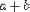<MINUS/> subtraction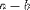<TIMES/> multiplication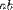<OVER/> division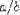<EXP/> "exponentiation"
<EXPR><EXP/><APPLY/>x</EXPR><POWER/> "to the power of"
<EXPR>x<POWER/>3</EXPR>

<DIV> "division modulo base"
<DIV>a<MOD/>N</DIV>

<REM> "remainder modulo base"
<REM>a<MOD/>N</REM>

<FACTORIAL> factorial
<FACTORIAL>n</FACTORIAL><MIN> minimum
<MIN>A</MIN><MIN> minimum
<MIN>
x <ST/> x <IN/>
<SET> x <ST/>
<E>
<EXPR>x<POWER>3</EXPR>
<LT/>
&Pi;
</E>
</SET>
</MIN><MAX> maximum
<MAX>A</MAX>### 4.2.3 Relations

TagDescriptionSample Rendering
<EQ/> equals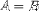<EXPR>A<EQ/>B</EXPR>
<NEQ/> not equal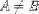<EXPR>A<NEQ/>B</EXPR>
<GT/> greater than<EXPR>A<GT/>B</EXPR>
<LT/> less than<EXPR>A<LT/>B</EXPR>
<GEQ/> greater than or equal<EXPR>A<GEQ/>B</EXPR>
<LEQ/> less than or equal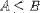<EXPR>A<LEQ/>B</EXPR>

### 4.2.4 Calculus

TagDescriptionSample Rendering
<LN> natural logarithm<LN>a</LN>
<LOG> logarithm;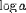<LOG>a</LOG>
use <DEGREE> for base; (see notes)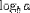<LOG>
a
DEGREE>b</DEGREE>
</LOG>
<INT> integral; (see notes)<INT>
<LOWLIMIT>0</LOWLIMIT>
<UPLIMIT>a</UPLIMIT>
<EXPR>x<POWER/>n</EXPR>
<BVAR>x</BVAR>
</INT>

<DIFF/> derivative, differentiation<EXPR>
<DIFF/>
f
<BVAR>x</BVAR>
</EXPR>

<PARTIALDIFF/> partial derivative<EXPR>
<PARTIALDIFF/>
f
<BVAR>x</BVAR>
</EXPR>

<TOTALDIFF/> total derivative<EXPR>
<TOTALDIFF/>
f
<BVAR>x</BVAR>
</EXPR>

<LOWLIMIT> lower limit of integral; (see notes)
<UPLIMIT> upper limit of integral; (see notes)
<BVAR> bound variable; (see notes)
<DEGREE> holds the n in "nth derivative"; (see notes)<EXPR>
<DIFF/>
f
<BVAR>x</BVAR>
<DEGREE>n</DEGREE>
</EXPR>


#### Notes on Calculus Elements

INT
The INT element uses the auxiliary qualifier elements UPLIMIT, LOWLIMIT and BVAR the limits of integration, the variable of integration. These optional elements may appear at any position inside the SUM or PRODUCT element. By default, the upper and lower limits are rendered in their usual positions, and the bound variable is rendered with a small space and the letter "d" in front of it.
BVAR
The BVAR element specifies a "bound variable". In different contexts, this means different things. In an integral, it specifies what integral is being integrated. In a derivative, it indicates which variable is being differentiated. The BVAR element is also used with sums and products.
LOWLIMIT and UPLIMIT
These elements specify lower and upper limits. In different contexts, this means different things. In an integral, they specify the limits of integration. These elements are also used to specify the limits of an index for sums and products, and also to specify a limiting value for a variable in a limit.
DEGREE
There are a number basic mathematical constructs which come in families, such as derivatives, moments, and logarithms to various bases. Rather than introduce special tags for each of these families, MathML uses a single general construct, the DEGREE element.

### 4.2.5 Theory of Sets

TagDescriptionSample Rendering
<SET> set<SET>
z <ST/>
<E>
<EXPR>F<APPLY/>z</EXPR>
<EQ/>0
</E>
</SET>

<UNION/> union (join)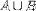<EXPR>
A
<UNION/>
B
</EXPR>

<INTERSECT/> intersection (meet)<EXPR>
A
<INTERSECTION/>
B
</EXPR>
<IN/> is in, or is an element of a set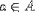<EXPR>
a
<IN/>
A
</EXPR>

<NOTIN/> is not in<EXPR>
a
<NOTIN/>
A
</EXPR>
<SUBSET/> is a subset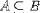<EXPR>
A
<SUBSET/>
B
</EXPR>
<PRSUBSET/> is a proper subset<EXPR>
a
<PRSUBSET/>
A
</EXPR>
<NOTPRSUBSET/> is not a proper subset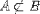<EXPR>
a
<NOTPRSUBSET/>
A
</EXPR>

### 4.2.6 Sequences and Series

TagDescriptionSample Rendering
<SUM> sum; (see notes)<SUM>
<LOWLIMIT>1</LOWLIMIT>
<UPLIMIT>N</UPLIMIT>
<BVAR>i</BVAR>
<EXPR>
<MSUB>
<MI>a</MI>
<MI>i</MI>
</MSUB>
</EXPR>
</SUM>
<PRODUCT> product; (see notes)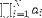<PRODUCT>
<LOWLIMIT>1</LOWLIMIT>
<UPLIMIT>N</UPLIMIT>
<BVAR>i</BVAR>
<EXPR>
<MSUB>
<MI>a</MI>
<MI>i</MI>
</MSUB>
</EXPR>
</PRODUCT>
<LIMIT> limit; (see notes)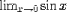<LIMIT>
<LOWLIMIT>x<TENDSTO/>0</LOWLIMIT>
<EXPR><SIN/>x</EXPR>
</LIMIT>

<TENDSTO/> tends to

#### Notes on Sequences and Series Content Schema:

SUM and PRODUCT
These elements, like the INT element, utilize the auxiliary qualifier elements UPLIMIT, LOWLIMIT and BVAR. These optional elements may appear at any position inside the SUM or PRODUCT element.
LIMIT
The LIMIT element also accepts the auxiliary qualifier element LOWLIMIT.

### 4.2.7 Trigonometry

We just list the names of the common functions for their interpretations should be clear.



### 4.2.8 Statistics

TagDescriptionSample Rendering
<MEAN> mean or average<MEAN>X</MEAN>
<SDEV> standard deviation<SDEV>X</SDEV>
<VAR> variance<VAR>X</VAR>
<MEDIAN> median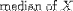<MEDIAN>X</MEDIAN>
<MODE> mode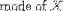<MODE>X</MODE>
<MOMENT> use <DEGREE> for the nin "nth moment"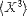<MOMENT>
X
<DEGREE>3<DEGREE>
</MOMENT>


### 4.2.9 Linear Algebra

TagDescriptionSample Rendering
<VECTOR> vector; see notes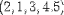<VECTOR>
2<SEP/>1<SEP/>3<SEP/>4.5
</VECTOR>
<MATRIX> matrix
<MATRIXROW> matrix row; see notes<E>
A<EQ/>
<MATRIX>
<MATRIXROW>
0<SEP/>1<SEP/>0
</MATRIXROW>
<MATRIXROW>
0<SEP/>0<SEP/>1
</MATRIXROW>
<MATRIXROW>
1<SEP/>0<SEP/>0
</MATRIXROW>
</MATRIX>
</E>
<MATRIXINVERSE> matrix inverse<MATRIXINVERSE>A</MATRIXINVERSE>
<DETERMINANT> determinant<DETERMINANT>A</DETERMINANT>

#### Notes on Linear Algebra Elements:

VECTOR and MATRIXROW
These elements both contain their entries as content. When the entries are ordinary data (#PCDATA) it is necessary to use a separator, in order to specify where one entry ends and another begins. In MathML, the SEP element is used. When the entries are actually valid MathML expressions, then no separator is needed. For example, if a MATRIXROW contains of a sequence of fully delimited EXPRS, the SEP elements may be omitted.

## 4.3 Syntax and Semantics

### 4.3.1 The Semantics of "Syntax" and the Syntax of <SEMANTICS>

The use of content rather than presentation tagging for mathematics is sometimes referred to as "semantic tagging" [BUS1]. The parse-tree of a fully bracketed MathML content tagged element structure corresponds directly to the expression-tree of the underlying mathematical expression. We therefore regard the content tagging itself as encoding the syntax of the mathematical expression. This is, in general, sufficient to obtain some rendering and even some symbolic manipulation (e.g., polynomial factorization).

However, even in such apparently simple expressions as X + Y, some additional information may be required for applications such as computer algebra. Are X and Y integers,or functions, etc.? What field's addition does the 'plus' represent? This additional information is referred to as Semantic Mapping. In MathML, it can be provided by the SEMANTICS and SEMINFO elements.

The SEMANTICS element expects up to three child elements. The first is the element (which may itself be a complex element structure) for which this additional semantic information is being defined. The second child is an optional separator. The third, optional, child is the SEMINFO element which contains the detailed semantic information:

<SEMANTICS>
<MathML Expression>
[<ST/>]
<SEMINFO> ... semantic data ... </SEMINFO>
</SEMANTICS>

The SEMINFO element is a container for arbitrary data. This data may be in the form of text, computer algebra encodings, C programs, or whatever a processing application expects. If the additional semantic information is contained in a well-formed XML element, as is the case for OpenMath SGML encoding, than this element can simply replace the placeholder SEMINFO element. For Example:
<SEMANTICS>
<EXPR> X <PLUS/> Y </EXPR>
<OM_APP>..</OM_APP>
</SEMANTICS>

where <OM_APP>..</OM_APP> are the elements defining the additional semantic information.

The SEMANTICS tags also accepts a "SEMTYPE" attribute for use by external processing applications. One use might be a URL for a semantic context dictionary, for example. Since the semantic mapping information might in some cases be provided entirely by the "SEMTYPE" attribute, the SEMINFO element is optional.

Of course, providing an explicit semantic mapping at all is optional, and in general would only be provided where there is some requirement to process or manipulate the underlying mathematics.

### 4.3.2 Semantic Mappings

Although semantic mappings can easily be provided by various proprietary, or highly specialized encodings, there are no widely available, non-proprietary standard semantic mapping schemes. In part to address this need, the goal of the OpenMath effort is to provide a platform-independent, vendor-neutral standard for the exchange of mathematical objects between applications. Such mathematical objects include semantic mapping information. The OpenMath group has defined an SGML syntax for the encoding of this information [OM]. This element set could provide the basis of one SEMINFO element set.

Part of the attraction of this mechanism is that the OpenMath syntax is SGML specified, so that the whole expression is checkable by a DTD-based parser.